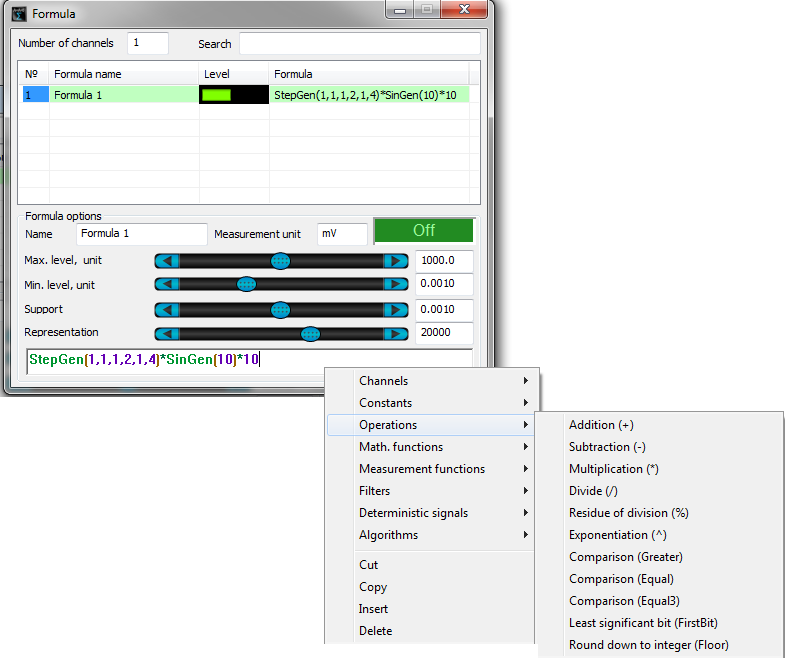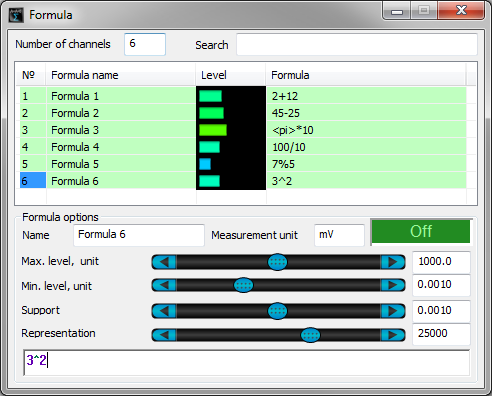# Operations

Formula program

The figure below shows “Operations” menu of Formula program.“Substraction (–)” – difference of channels, constants, equation results.

“Multiplication (*)” – product of channels, constants, equation results.

“Division (/)” – quotient of channels, constants, equation results.

“Residue of division (%)” – remainder of the division of channels, constants, equation results.

“Exponentiation (^)” – exponentiation of channels, constants, equation results.

“Inversion (!)”, “Module (|)”, “Comparison (Greater)”, “Comparison (Equal)”, “Comparison (Equal3)”, “Least significant bit (FirstBit)” – the components are described in the examples below.

The figures below show examples of basic math operations “+”, “-“, “*”, “/”, “%” and “^”. For illustration purposes, there are used numbers (constants), however, these operations are also applicable to channels (instant signal values) and equation results. The values of Formula channels are measured by DC voltmeters since in this particular example the calculation result is represented by a constant.«Inversion (!)» – unary postfix inversion operator, equal to multiplication by (-1).

«Module (|)» – unary postfix module operator: sets positive sign for all values.

The figure below shows an example of inversion and module operation. As an example, we use TriGen function (saw-shaped signal). The oscillograph depicts source signal (Formula 1), an inverted signal (Formula 2), and a modulus signal (Formula 3).Greater (а1, а2) – comparison operation, returns 1 if the first operand is greater than the second one and 0 in other cases.

Equal (а1, а2, const) – comparison operation for equality, if the modulus difference between the two operands is less than the const value, the operation returns 1 and 0 – in other cases.

Equal3 (а1, а2, const) – comparison operation for equality, if the difference between the first and the second operands is greater than the const value, the operation returns 1, if the difference is less than the const value, the operation returns -1, in other cases – 0.

Operations Greater, Equal, Equal3 are perfectly suitable for functions of threshold detection and creation of various regulators. As the operands, one can use channels (the operations will be performed with instant signal values), constants and equations (the operations will be performed with calculations results).

The figure shows an example of Greater function operation. The value of “Channel 1” signal is 1,5 V in the case if “Thermocouple1” value is less than 90. It is also possible to establish a system of constant temperature maintenance. Connect a relay to the DAC output for the control of current level at the heating element, start the program Signal generator, configure it for the control of “Channel ” signal.The figures below show an example of Formula program application, in particular – Equal and Equal3 operations principles. For illustration purposes, there are 3 channels used in this example. The first channel, “Difference“, is used to calculate the difference between “Control sensor” and “Sensor” measuring channels’ instant values. The second channel represents the result of Equal operation, the third channel displays the result of Equal3 operation.### You may also be interested in…

Delivery terms: CIP, CPT or DAP. Customs clearance and delivery is carried out by the "Cordely" custom company (included in the price of the goods). 1992 - 2023 ZETLAB Company